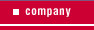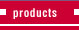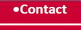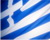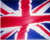air conditioning heat pumps ventilation air curtains solar installation-maintenance

Calculate Air Conditioner
(split - multi -vrf)

Calculate your space properly to choose the right air conditioner, earning money from long term power consumption and longer life of your air conditioner

See video installation of air conditioners in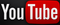View Photo Installation of air conditionsWe undertake systems and preinstallation air conditioning and ventilation systems in buildings and constructions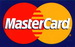# Calculate Air Conditioner The most common way of calculating btu/h needed a space is the square meter (m²). That is, up to 20m² - 9000btu/h, up to 30m² - 12000btu/h, up to 40m² - 18000btu/h, up to 50m² - 24000btu/h. There is just so. The correct calculation should include the amount of space you want to cover. We know that to cubic meters (m³). Length times the width of a room gives the area in square meters (L x W = m²). The square that we calculated by multiplying the height of the room and gives us all the cubic feet (L x W x H = m³) or (m² x H = m³) Now, if we know m³ multiply the number 170 for a room with minimal losses, with the number 180 or 190 for a room with average losses and the number 200 for a room with several casualties. The numbers 170 ~ 200 denote the btu/m³. Losses include, for example masonry, altitude you find the building's windows, if unprotected high plate on the roof, etc. Example: Suppose we have a space / room with minimal losses of 30m². Normally the prevalent fashion needs an air conditioner 12000btu / h. When the height of the room is normal, is 2,50m is just so. This follows from the correct calculation: 30m² x 2,50 m = 75m³ x 170 = 12.750btu/h. Suppose now that the same space / room height is 3m. Look how the data changes: 30m² x 3m = 90m³ x 170 = 15.300btu/h. To the air conditioner 12000btu now is small for the space / room. And if you find in the city center, rooftop with unprotected high plate to the roof and walls with minimal insulation (the majority of buildings) then the data are: 30m ² x 3m = 90m ³ x 200 = 18.000btu / h To the air conditioner 12000btu now is unsuitable for the space / room. Easily find that our criterion for proper choice of air conditioner should not only be the size. Cubist your space more accurately measured. Certainly play a role and other factors, but in 90% of cases, this rule applies. Of course the examples mentioned are indicative and in no way replaces the study of engineering.      Now if you want to convert btu, in Watt or kcal click the following operations:      1btu / 3,96 = kcal / h      1btu / 3,412 = Watt / h      example      12.000btu / 3,96 = 3.030 kcal / h      12.000btu / 3,412 = 3.517 Watt / h      If you now want to convert Watt in kcal:      1.000Watt x 860,42 = 860,42 kcal / h      or      30.000 kcal / h / 860,42 = 34.866 Watt / h Amicably Sotiris Karkas Mechanical Refrigeration

See what is included in the installation

We undertake installations of air conditioners split, central and central systems, VRF

Photos